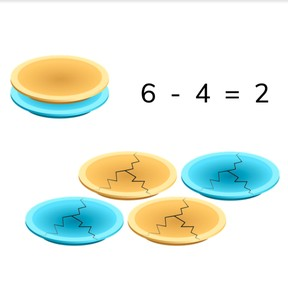Recognizing subtraction problems to 10

# Recognizing subtraction problems to 108,000 schools use Gynzy92,000 teachers use Gynzy1,600,000 students use Gynzy

## General

Students learn to use an image to help understand a subtraction problem.

1.OA.A

## Relevance

Discuss with students that it is useful if you can use images to represent mathematical problems. You can use the images to help understand the problem and use them to help learn how to subtract.

## Introduction

Show a selection of animals on the interactive whiteboard. Have the students count how many of each kind of animal there are. Ask students if they know any clever ways to count the animals. Say that you can cross off the animals as you count them to make sure you don't miss any or count them twice. When you have an answer, erase the grey square to check the answer.

## Development

Show ice cubes on the interactive whiteboard. Some ice cubes are melted, and others are still frozen. First count how many ice cubes there are in total and then count how many are melted. There are 10 ice cubes in total, and 1 has melted. If you take the one melted ice cube away from the others, you have 9 left. The subtraction problem this represents is then 10 - 1. Discuss all the variations of ice cubes/melted cubes with your students and be sure to show what the matching subtraction problem is.
Next show students the matches. Count the total number of matches, there are 6. Tell students that 2 matches have been struck and are taken away. Emphasize that the - (minus sign) means to take away because the second number (subtrahend) is taken from the first, or total number (minuend).
Next show the notepads, and note that some of the notepads are out of paper. Ask students to determine which subtraction problem is shown by the image. Erase the grey boxes to show the answer. Next students must drag apples and apple cores to form an image that matches the given subtraction problem.
Using the matches, discuss with students that when they are able to form the subtraction problem using the image, they can also solve for the difference by taking the second number (subtrahend) away from the first number (minuend). The difference is the number that is left. If you take away the burnt matches from the total number of matches, you determine the difference. Check to see that students can find the difference in an exercise with paint tubes.

Check that students are able to use an image to determine and solve subtraction problems by asking the following questions:
- How do you know which numbers are part of a subtraction problem?
- What do you do if you want to determine how many objects are left if you take some away?
- What is the first number of a subtraction problem? (what does it represent)
- How do you determine which number is second in a subtraction problem?

## Guided practice

Students first practice recognizing subtraction problems from given answers. Then they must determine which subtraction problem matches a given image, and finally students are asked to determine the subtraction problem and difference of a given image.

## Closing

Discuss with students that it is important to be able to use images to understand subtraction problems to help understand how subtraction works. Check that students are able to form subtraction problems based on a given image. Ask them to write their answers on a sheet of paper and hold up their answers so you can check their work.

## Teaching tips

Students who have difficulty recognizing subtraction from given images can make use of manipulatives, like MAB blocks. Set out a group of blocks and have the student count out the blocks. Then remove a number of the blocks and ask the student to count that amount, and finally to count how many blocks are left.

## Instruction materials

Optional: manipulatives or MAB blocks

### The online teaching platform for interactive whiteboards and displays in schools

• Save time building lessons

• Manage the classroom more efficiently

• Increase student engagement